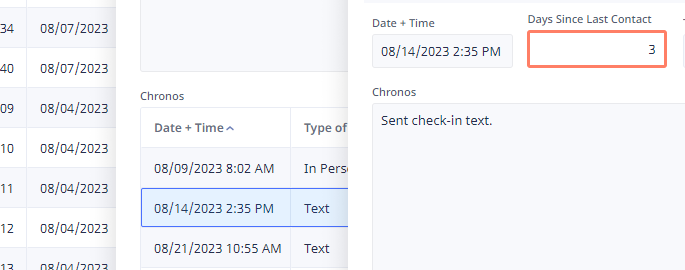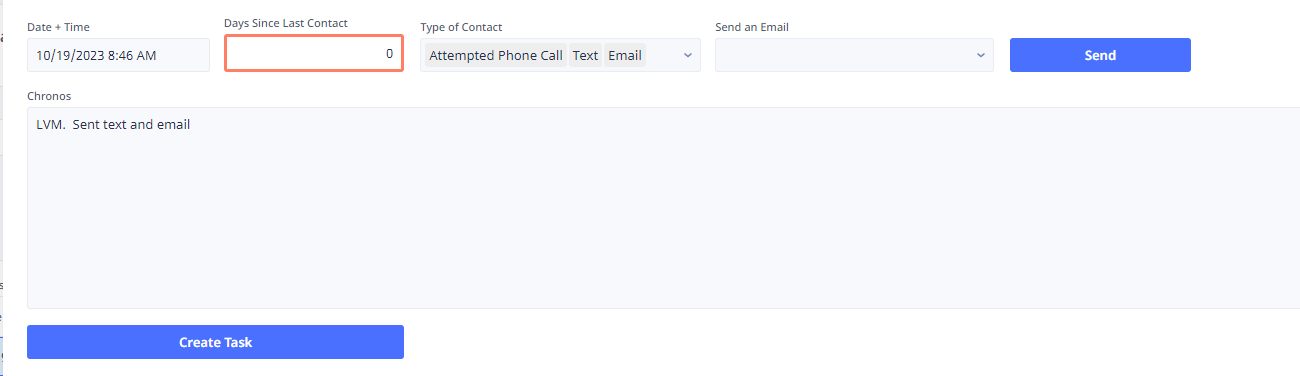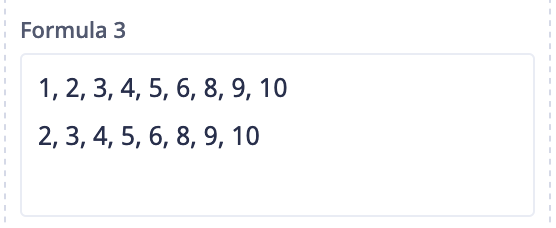0

# Need Script for calculating workdays between 1st record, 2nd record, so on.

Ok, Thanks to  I am able to calculate the working days since a previous record for a client.with the following script

``````let t := this;
let xBase := ('Clark Leads'.Chronos order by 'Date + Time');
let xCurIndex := index(xBase, t);
if xCurIndex = 0 then
0
else
let xPrev := item(xBase, xCurIndex - 1);
workdays(xPrev.date('Date + Time'), t.date('Date + Time')) - 1
end
``````

However, for accountability purposes, I have started another Sub Table that Provides Statistics for Avg (prefer median) working days for all client Chono Records.  I have the overall average for the correct statuses I need using the following script:

``````do as server
avg((select Leads where 'Lead Student Type' = 2 and (Status = 1 or Status = 2 or Status = 12 or Status = 13)).'Days Since Last Contact')
end
``````

Now I want to separate the overall Stats for the amount of working days between each contact.  For instance, I want the avg (prefer median) of working days between the 1st contact (first chrono record for that client) and the second contact (second chrono record for that client).  I will continue replicating this script all the way up to between their 4th and 5th contact.

I am keeping these stats on a subtable with the Parent Table being Leads.

Any help is greatly appreciated.

## 15replies• Fred
• 3 mths ago
• Reported - view
Dave Irving said:
For instance, I want the avg (prefer median) of working days between the 1st contact (first chrono record for that client) and the second contact (second chrono record for that client).

I'm unsure what you want here.

You have the number of days between chrono record for a parent client record. I don't know how you can find the average between 1st and 2nd contact of an individual client record since you only have 1 data set for 1st contact and one data set for 2nd.

Or are you wanting to find the average number of days between all 1st and 2nd contacts for all clients? This you can do since you will now have more than 1 number to average.

You have the number of days already figured out. You can just find the right filter then avg('Days Since Last Contact').

• Dave_Irving
• 3 mths ago
• Reported - view

Yes, I need the avg for All Leads meeting a certain filter.  But I need averages for each situation.  The avg of days between 1st Chrono record and second, and so forth as described above.  This, I need the script to be able to sort each individuals chronos records by date...avg the days between 1st ordered record by date and 2nd record by date.  It's more complicated then just doing the average of days between all records, because it needs to split it up.

• Fred
• 3 mths ago
• Reported - view
said:
This, I need the script to be able to sort each individuals chronos records by date...avg the days between 1st ordered record by date and 2nd record by date.

You have the field Days Since Last Contact which finds the number of days between the current record in Chronos and the previous record when ordered by a date field. So if we can get the 2nd record in Chronos for each record in 'Clark Leads' then take the average of 'Days Since Last Contact' we will get what you want?

If that is true then this should do it:

``````let x := (select 'Clark Leads');
let y := for loop1 in x do
item(loop1.Chronos order by 'Date + Time', 1)
end;
avg(y.'Days Since Last Contact')
``````

Line 1, gets all the records in 'Clark Leads', if that is not the table name then change it to match your table name. We start here because we can use the relatonship to Chronos to find the 2nd record in Line 3.

Lines 2 - 4, is the for loop that will go through each record in 'Clark Leads'. Then it will take all related records in the Chronos relationship field order it by 'Date + Time' field and then find the 2nd record in the array of records. Since Ninox starts all list with a 0, the 2nd record is number 1.

Line 5, then finds the average of the field 'Days Since Lat Contact'.

• Dave_Irving
• 3 mths ago
• Reported - view

Sorry Fred, I was gone for vacation.  However, I had tried this and it returns a value of 0.  I did do sum just to check to see if it was pointing in the right direction and still received 0.  Code as follows:

``````let x := (select Leads);
let y := for loop1 in x do
item(loop1.Chronos order by 'Date + Time', 1)
end;
avg(y.'Days Since Last Contact')
``````
• Fred
• 3 mths ago
• Reported - view

Ok, now we do a bit of troubleshooting.

1) verify that "y" has the record Ids that match. You can do the following in another field:

``````let x := (select Leads);
let y := for loop1 in x do
item(loop1.Chronos order by 'Date + Time', 1)
end;
#{avg(y.'Days Since Last Contact')}#;
concat(y)
``````

That should give you the record Id of all records in Chronos. If those are correct then you should look in those records to see if you have any data in the field 'Days Since Last Contact'. If there is data in those fields then to verify you can change the field to:

``````let x := (select Leads);
let y := for loop1 in x do
item(loop1.Chronos order by 'Date + Time', 1)
end;
#{avg(y.'Days Since Last Contact')}#;
concat(y.'Days Since Last Contact')
``````

That should show you the individual numbers in the field 'Days Since Last Contact' for each record.

In one of the above instances you should be able to figure out where the issue is.

• Dave_Irving
• 3 mths ago
• Reported - view

it actually did work.  Just took some time for the server to catch up !!!  I modified the code to allow me to filter it also for dates so I can monitor trends.

``````let curRec := this;
let xStart := 'Start Date';
let xEnd := 'End Date';
where 'Lead Student Type' = 2 and 'Lead Entered Date' >= xStart and
let y := for loop1 in x do
item(loop1.Chronos order by 'Date + Time', 1)
end;
avg(y.'Days Since Last Contact')
``````
• John_Halls
• 3 mths ago
• Reported - view

Hi Dave The above code will run in the Console so you can see what results you are getting. Try a simple

``````let x := (select Leads);
let y := for loop1 in x do
item(loop1.Chronos order by 'Date + Time', 1)
end;
y
``````

and see what you get.

• Dave_Irving
• 1 mth ago
• Reported - view

, Hey Fred.  I am circling back around to this.  However, now I want to do the avg of all workingdays between each Chrono.  However, it has to not count that first Chrono in the record because that value is 0 and throws the avg off since there are obviously a couple thousand chrono records with 0.  Any advice while modifying the code here?• Fred
• 3 mths ago
• Reported - view

For median you can try something like:

``````let mynumber := ((select 'My table') order by Number).Number;
let mycnt := cnt(mynumber);
let mymedian := if even(mycnt) then
(item(mynumber, mycnt / 2 - 1) + item(mynumber, mycnt / 2)) / 2
else
item(mynumber, mycnt / 2)
end;
mymedian``````

Since Ninox starts with 0 you have subtract 1 after you divide the count so you can get the correct value. For example if you have 4 numbers (2,5,8,12). The count is 4 and half of that is 2. If you ask for the value of the item 2 Ninox will return 8, since 2 is 0.

• Fred
• 1 mth ago
• Reported - view
• Dave_Irving
• 1 mth ago
• Reported - view

``````let curRec := this;
let xStart := 'Start Date';
let xEnd := 'End Date';
where 'Lead Student Type' = 2 and 'Lead Entered Date' >= xStart and
let y := for loop1 in x do
item(loop1.Chronos order by 'Date + Time', 1)
end;
avg(y.'Days Since Last Contact')
``````

For this, we won't need to put the Chrono records in order, and instead of the second record (1), we need to include all Chrono records for qualifying leads.  However, still need to not include the 1st chrono record for all leads so we can remove the 0 values from the equation.

• Fred
• 1 mth ago
• Reported - view

Approaching this another way, can the code for 'Days Since Last Contact' be changed to  use a null value instead of 0? That way you can include it in any function and it won't be counted.

• Fred
• 1 mth ago
• Reported - view
Dave Irving said:
However, still need to not include the 1st chrono record for all leads so we can remove the 0 values from the equation.

This code might work. I think we still need to sort so we can make sure to drop the proper first record.

``````let curRec := this;
let xStart := 'Start Date';
let xEnd := 'End Date';
where 'Lead Student Type' = 2 and 'Lead Entered Date' >= xStart and
let y := for loop1 in range(1,count(x)) do
item(x,loop1)
end;
avg(y.'Days Since Last Contact')``````

Since Ninox starts all arrays with 0, we create a range starting with 1 and we automagically have an array that drops the first instance.

Here is what it looks like in my test:The first line is all the records in a table. The second line drops the first instance of the returned array.

Here is the code:

``````let x := (select Table1);
let y := for loop1 in range(1, count(x)) do
item(x, loop1)
end;
concat(x) +
"
" +
concat(y)
``````
• Dave_Irving
• 1 mth ago
• Reported - view

, I like your first idea of making the "Days Since Last Contact" value null if it is the first chrono record for that lead. Here is the script for that "Days Since Last Contact" field.  I replaced 0 with null, but that broke it.

``````let t := this;
let xBase := ('Clark Leads'.Chronos order by 'Date + Time');
let xCurIndex := index(xBase, t);
if xCurIndex = 0 then
0
else
let xPrev := item(xBase, xCurIndex - 1);
workdays(xPrev.date('Date + Time'), t.date('Date + Time')) - 1 -
cnt(select '2023-2024 Holiday Schedule' where Date >= xPrev.date('Date + Time') and Date <= t.date('Date + Time'))
end
``````
• Fred
• 1 mth ago
• Reported - view
said:
Here is the script for that "Days Since Last Contact" field.  I replaced 0 with null, but that broke it.

So if you want xCurIndex to be null then you have to think the opposite. Instead of looking for = 0 do the opposite and have no "else". Like:

``````let t := this;
let xBase := ('Clark Leads'.Chronos order by 'Date + Time');
let xCurIndex := index(xBase, t);
if xCurIndex != 0 then
let xPrev := item(xBase, xCurIndex - 1);
workdays(xPrev.date('Date + Time'), t.date('Date + Time')) - 1 -
cnt(select '2023-2024 Holiday Schedule' where Date >= xPrev.date('Date + Time') and Date <= t.date('Date + Time'))
end
``````

Zero is not null. Zero is a value, as you find out when you do an average. It is included.

## Content aside

• 1 mth agoLast active
• 15Replies
• 113Views
• 3 Following